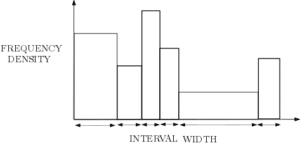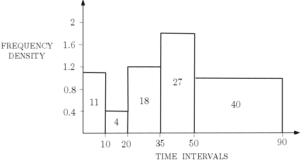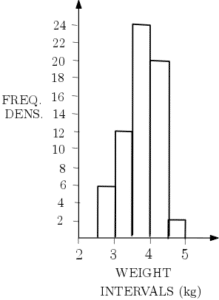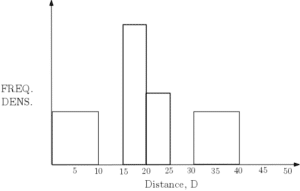# Histograms

Histograms provide a useful way to display data and its distribution. Students often get confused between histograms and bar charts. However, histograms and bar charts are different in a number of ways. Firstly, histograms are used to present continuous grouped data whereas bar charts are used to display ordinal, nominal or discrete data. Click here to see some examples of bar charts. Secondly, bar charts are usually used to compare data whereas a histogram shows the distribution of the data. Note that you may be required to produce or interpret histograms for a given dataset but you may also be asked to do it for a dataset that you are already familiar with. See more on this.

The most important aspect of histograms is that they are not plotted against frequency, they are plotted against frequency density. This means that the frequency is represented in the area of the bar.Since the area of a rectangle is width multiplied by height, we have:

FREQUENCY = FREQUENCY DENSITY $\times$ INTERVAL WIDTH

See the worked example for an application of this. Note that the area may be exactly the frequency but it is also possible to use proportional areas. In these cases:

FREQUENCY = $k\times$ AREA

where $k\ne 1$ – see Example 1. If the interval widths are uniform, a frequency polygon (see more on Frequency Polygons) can be added to a histogram by joining up the midpoints of the top of each bar of the histogram with straight lines. See Example 1d. Histograms also provide a convenient way to look at the ‘spread’ of data (see Measures of Variation) and connect to probability distributions. See the Box Plots page (including the example) for more on this.

## Histogram Examples

The following table shows the times 100 people waited in a queue for KFC at a specific store in the first hour of reopening during the CoronaVirus lockdown in 2020.

 Queue Time, t (minutes) Frequency $0\leq t\hspace{3pt}<\hspace{3pt} 10$ 11 $10\leq t\hspace{3pt}<\hspace{3pt} 20$ 4 $20\leq t\hspace{3pt}<\hspace{3pt} 35$ 18 $35\leq t\hspace{3pt}<\hspace{3pt} 50$ 27 $50\leq t\hspace{3pt}<\hspace{3pt} 90$ 40

In order to create a histogram to display this data, we must calculate the frequency densities. That is, we need to find the height of each bar. Once we know the largest frequency density, we can choose the vertical scale for our histogram. Using the above formula:

FREQUENCY DENSITY = FREQUENCY $\div$ INTERVAL WIDTH

The interval widths and frequency densities are as follows:

 Interval Width Frequency Density 10 1.1 10 0.4 15 1.2 15 1.8 40 1

These columns are often added to the original table. The largest frequency density is 1.8 and so we choose the vertical scale accordingly. The histogram is constructed as follows.Click here for some more statistical analysis using this example (Variance and Standard Deviation – Example 2).

The following histogram shows the masses of some domestic housecats in kilograms:1. Justify why a histogram might be used for this data.
2. Given that the number of cats between 3kg and 3.5 kg is 18, construct the frequency table that accompanies this histogram. How many cats have been weighed in total?
3. Estimate how many cats weight more than 3.8kg.
4. Add the associated frequency polygon to this histogram.

The incomplete histogram and frequency table for the distance travelled to get to work by 100 employees of a given company are as follows:Distance, D (km) Frequency $0\leq D\hspace{3pt}<\hspace{3pt} 10$ $10\leq D\hspace{3pt}<\hspace{3pt} 15$ 15 $15\leq D\hspace{3pt}<\hspace{3pt} 20$ $20\leq D\hspace{3pt}<\hspace{3pt} 25$ 10 $25\leq D\hspace{3pt}<\hspace{3pt} 30$ 20 $30\leq D\hspace{3pt}<\hspace{3pt} 40$ $40\leq D\hspace{3pt}<\hspace{3pt}50$
1. Complete the histogram and the frequency table.
2. Using midpoints, find an estimate for the mean time travelled (to one decimal place).
3. What is the median distance travelled?
4. Using linear interpolation, find estimates for the lower quartile Q1 and the upper quartile Q3.